# PSAT Math : How to multiply integers

## Example Questions

### Example Question #1 : Operations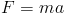in physics where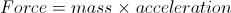.

If the mass is increased by 3 three times the original and the acceleration is increased by 7 times the original, how many times greater is the new force than the original force?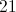Explanation:

We simply multiply the new mass by the new acceleration to obtain a new force that is 21 times greater than the original.

### Example Question #2 : Basic Operations

An office wants to buy 22 computers at $900 each. The budget is$20,000 and the tax on computers is 9%. How many computers can the office afford?Explanation:

The office can only afford 20 computers.

1.09 * 900 = \$981 is the actual price of each computer, with tax.

Divide:

20,000/981 = 20.39

Since the office cannot purchase a partial computer, we round down to 20 computers.

### Example Question #1 : How To Multiply Integers

What property of arithmetic is demonstrated below?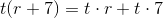Associative

Symmetric

Commutative

Distributive

Transitive

Distributive

Explanation:

The statements demonstrates that the product of a number and a sum can be found by multiplying the number by each of the addends and adding the products. This is the distributive property of multiplication over addition.

### Example Question #2 : How To Multiply Integers

What property of arithmetic is demonstrated here?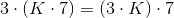Commutative

Associative

Transitive

Symmetric

Associative

Explanation:

The symbols express the idea that if three numbers are multiplied, the same product results regardless of which numbers are multiplied first. This is the associative property of multiplication.

### Example Question #3 : How To Multiply Integers

Multiply in modulo 8: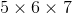None of the other responses is correct.Explanation:

In modulo 8 arithmetic, a number is congruent to the remainder of its division by 8.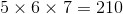and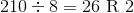so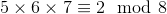making the correct response.